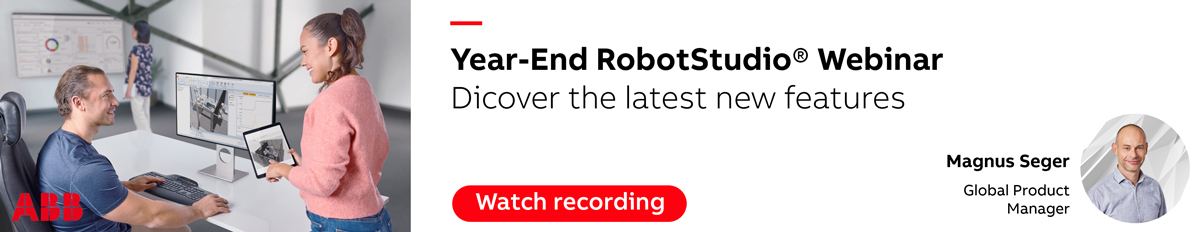# Conveyor tracking without the software

I want to achieve the conveyor tracking function without the use of the standard conveyor tracking software.... is this possible?? if yes then how do i go about it...
Robot Engineer

• If you want to use conveyor tracking with ABB robots, then ABB recommends you to have the conveyor tracking option in you robot system.

Henrik Berlin
ABB

i am trying this as an experiment...

i am using a timed interrupt to trigger a trap routine in which i am calculating the position offset using a simple velocity formula

Position offset = velocity x time

where,

Velocity 'V' = constant
time      't'  = instantaneous time

i am calculating the instantaneous time by starting a clock at the trigger of a "sync switch".
i stop this clock only at the end of the program (after completing the conveyor tracking operation).

the program is fairly simple ie., to form a rectangle on a plate which is moving on a conveyor.

i have tried this on other robots and it has worked quite well. so i know its possible on an ABB as well.

all this while i have assumed the velocity to be constant; but now i want to calculate the velocity as well as use the instantaneous velocity value in the above formula , to obtain a more accurate path.

Can anybody help me on this...

Robot Engineer